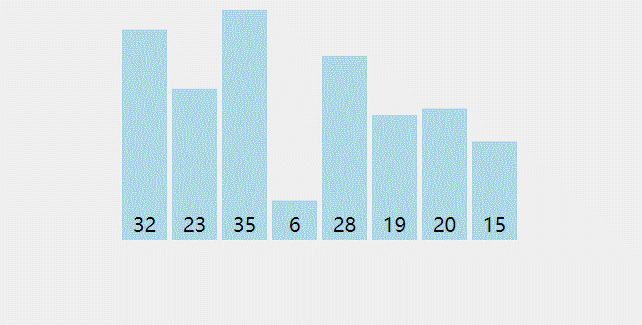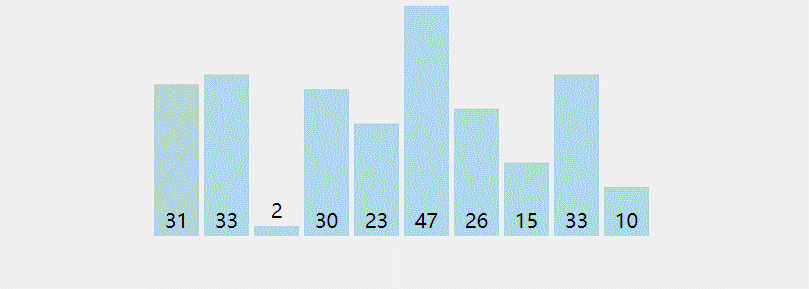Don't Panic.Steve

## 冒泡排序

1. 比较相邻的元素。如果第一个比第二个大，就交换他们两个。
2. 对每一对相邻元素作同样的工作，从开始第一对到结尾的最后一对。这步做完后，最后的元素会是最大的数。
3. 针对所有的元素重复以上的步骤，除了最后一个。
4. 持续每次对越来越少的元素重复上面的步骤，直到没有任何一对数字需要比较。

visualgo上模拟的动画如下C语言实现:

void BubbleSort(int a[],int length)
{
int i, j, temp;
for(i = 0; i <= length - 1; i++ )
{
for(j = 0; j < length; j++)
{
temp = a[j];
if(a[j] > a[j+1])
{
a[j] = a[j+1];
a[j+1] = temp;
}
}
}
}


## 插入排序

1. 从第一个元素开始，该元素可以认为已经被排序
2. 取出下一个元素，在已经排序的元素序列中从后向前扫描
3. 如果该元素（已排序）大于新元素，将该元素移到下一位置
4. 重复步骤3，直到找到已排序的元素小于或者等于新元素的位置
5. 将新元素插入到该位置后
6. 重复步骤2~5

visualgo上模拟的动画如下C语言实现

void insertion_sort(int a[], int len)
{
for (int i = 1, j = 0 ; i < len; ++i)    //对比len - 1次，直到最后只剩一个数
{
int temp = a[i];
for(j = i - 1; j >= 0 && a[j] > temp; j--)
{
a[j+1] = a[j];
}
a[j+1] = temp;
}
}